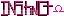-=+=- -=+=- -=+=- -=+=- -=+=- -=+=- -=+=- -=+=- -=+=- -=+=- -=+=- -=+=- -=+=- -=+=- -=+=- -=+=- -=+=- -=+=- -=+=- -=+=- -=+=- -=+=- -=+=- -=+=- -=+=- -=+=- -=+=- -=+=- -=+=- -=+=- (c) WidthPadding Industries 1987 0|614|0 -=+=- -=+=- -=+=- -=+=- -=+=- -=+=- -=+=- -=+=- -=+=- -=+=- -=+=- -=+=- -=+=- -=+=- -=+=- -=+=- -=+=- -=+=- -=+=- -=+=- -=+=- -=+=- -=+=- -=+=- -=+=- -=+=- -=+=- -=+=- -=+=- -=+=- Socoder -> Off Topic -> Hello! Posted : Saturday, 26 April 2008, 03:45 AmonMan this site layout and style sure brings back some memories. I've registered here after finding out about this site from syntaxbomb. There are a lot of old names here that I remember. Anyway, Hello to all! Regards AmonPosted : Saturday, 26 April 2008, 03:55 HoboBenHello! Welcome homePosted : Saturday, 26 April 2008, 04:14 JayenkaiWelcome back Amon!Posted : Saturday, 26 April 2008, 04:16 shroom_monkWelcome and Hello! Posted : Saturday, 26 April 2008, 04:18 AmonThanks Guys! I'm looking forward to being part of the community. Hope to see some good games and code posted. Thanks again!Posted : Saturday, 26 April 2008, 05:30 blanko1324You're back!Posted : Saturday, 26 April 2008, 06:54 HotshotWelcome and HellooooooooooooooooooooooooooooooPosted : Saturday, 26 April 2008, 07:59 PhoenixHappy birthday! We must've missed your last one. Posted : Saturday, 26 April 2008, 08:06 JL235heyPosted : Saturday, 26 April 2008, 08:17 DabzHowdy Amon, ya tinker!DabzPosted : Saturday, 26 April 2008, 08:38 Schererererwelcome home amon!Posted : Sunday, 27 April 2008, 16:29 MogOllddd name 'lo Amon.Posted : Monday, 28 April 2008, 05:25 Afr0'Lo there, ya old owl!